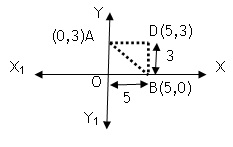Maths / Coordinate Geometry / Distance Formula

QUESTION

AOBD is a rectangle whose three vertices are vertices A(0,3), O(0,0) and B(5,0). The length of its diagonal is

 OPTIONS A. 5 B. 3 C. D. 4
Right Option : C

EXPLANATION
Explain TypeExplanation Content
Text

We know that, the adjacent of rectangle having angleUse Pythagoras theorem in

which is the required length of diagonal of rectangle.

TestimonialsSTUDENT FEEDBACK - Ayan ghosh C/o ABHYAS Academy
8th
My experience was very good with Abhyas academy. I am studying here from 6th class and I am satisfied by its results in my life. I improved a lot here ahead of school syllabus.